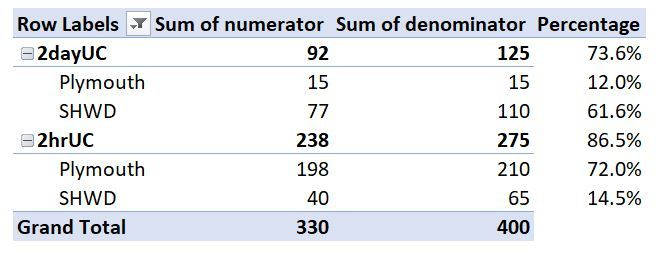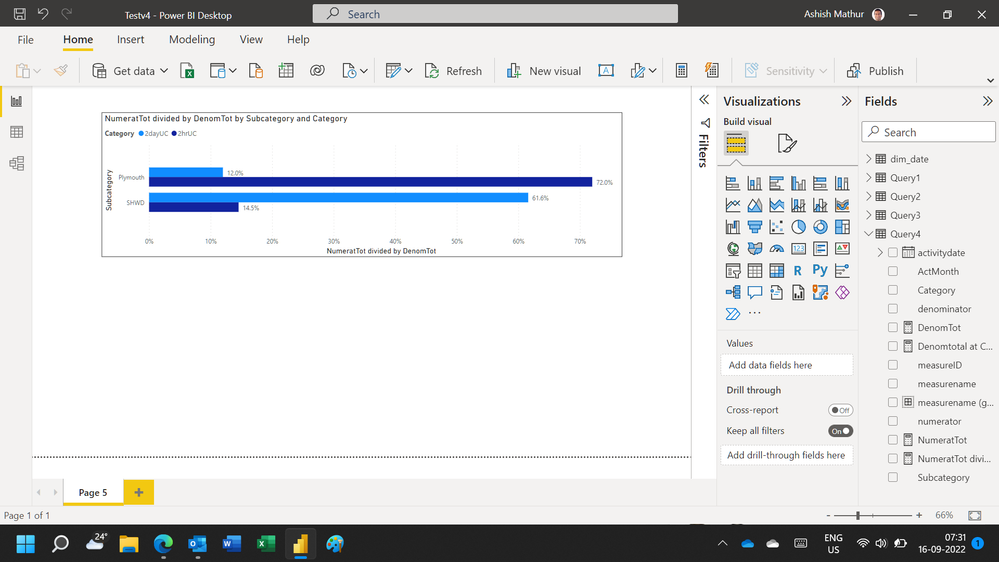cancel
Showing results for
Did you mean:Helper I

## how to divide subcategory sum by category total

Hi Guys,

Anyone know how I can create measures that give the percentage figures as shown in the pivot table below? The category totals are 125 and 275 and whenever i try to create a percentage total of each subcategory ie Plymouth or SHWD, it keeps dividing the subcategory numerator total by the respective subcategory denominator total (15, 110, 210 and 65). I need a measure that can divide the subcategory numerator total by the respective category denominator total instead (125 or 275) as shown on the pivot table. I have also included the pbix file and when you look at it, it shows the percentage figures for each subcategory when its numerator total is divided by the subcategory denominator total and this is not what i want to see. I need it to be abe to calculate the percentages just as it is on the pivot table.

Any help will be highly appreciated.pivot table

2 ACCEPTED SOLUTIONSSolution Specialist

Hi try this

Measure =

var cat =SELECTEDVALUE (category Column)

return

if(ISINSCOPE(sub-category Column),
sum of numberator / CALCULATE(sum of denominator, REMOVEFILTER(sub-category column), category Column = cat),
sum of numbertor/sum of denominator)Super User

Hi,

Hope this helps.Regards,
Ashish Mathur
http://www.ashishmathur.com
6 REPLIES 6Super User

Hi,

Hope this helps.Regards,
Ashish Mathur
http://www.ashishmathur.comHelper I

Thank you @Ashish_MathurSuper User

You are welcome.

Regards,
Ashish Mathur
http://www.ashishmathur.comSolution SpecialistSolution Specialist

Hi try this

Measure =

var cat =SELECTEDVALUE (category Column)

return

if(ISINSCOPE(sub-category Column),
sum of numberator / CALCULATE(sum of denominator, REMOVEFILTER(sub-category column), category Column = cat),
sum of numbertor/sum of denominator)Helper I

Thank you @HoangHugo